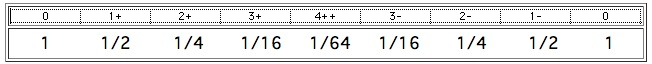# universal constant

Russell
"The Universal One divides His time by the periodicities of His inhalations and exhalations, which together make one universal constant of energy, and subdivides it into four exactly equal unit constants of energy. These periodicities, and tonal and mid-tonal subdivisions, measure the dimensions of His idea in the illusion of form, space, time, sex, temperature and other periodicities." Russell, The Universal One (underline added)
[see universal constant of energy]

Gravitation is the generative force and radiation is the degenerative force of the universal constant. These are the opposing powers which, within all mass, seek an equilibrium zone of pressure for the potential of that mass. [The Universal One, Book 02 - Chapter 12 - Gravitation and Radiation, page 141]Figure 9.12 - Scale of Locked Potentials over Time

"The seven tones of the universal constant are each removed, one from the other, the square of the distance to the next highest potential. The energy of each of the four units is exactly the same." [from chart "Universal Mathematics - Dimension Chart No. 5" of The Universal One]

THE SEVEN TONES OF THE UNIVERSAL CONSTANT ARE EACH REMOVED, ONE FROM THE OTHER, THE SQUARE OF THE DISTANCE TO THE NEXT HIGHEST POTENTIAL. THE ENERGY OF EACH OF THE FOUR UNITS IS EXACTLY EQUAL. [Dimension Chart 5]